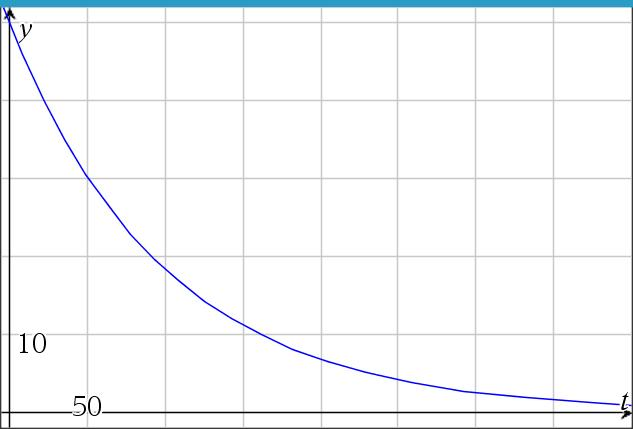# Brine mixture, case 1, inflow=outflow, pure water in

Up a level : Differential Equations
Previous page : A harder example of solving a linear first order equation
Next page : Brine mixture, case 2, inflow≠outflow, pure water inThe brine problem

We will have a look at the classical brine mixing problem.  In this we will have a tank full of brine (salt water) with some initial volume and amount of salt. We will have an inlet where brine with some constant concentration will enter at a constant rate, and an outlet where brine will flow out of the tank at some constant rate. We will assume that the brine we pour in will basically mix evenly with the brine in the tank. Our goal is to find out how the amount of salt in the tank varies over time.$\frac{{dy}}{{dt}} = {k_1}{r_1} - {k_2}{r_2}$

Here y=the amount of salt in the tank, k1 =the concentration of the inflowing brine, r1=the rate of the inflow, so some volume of brine per time, k2=the concentration of the out-flowing brine and r2=the rate of the outflow.

We can see that the units will match up since we have the rate of change of amount of salt per time unit, say kg/s, on the left-hand side, and${k_1}{r_1} - {k_2}r \Rightarrow \frac{{dy}}{{dV}}\frac{{dV}}{{dt}} = \frac{{dy}}{{dt}}$

on the right-hand side too.

The equation we started with is a very general equation, and it is true even k1, k2, r1 and r2 are changing over time. In our example all but r2 will be constants though.

We will look at a few cases in this and the next few pages.

Case 1r1= r2=r, k1=0, V=V0

We will thus have the same inflow as outflow rate, and the inflow will be pure water. This will give us the equation$\frac{{dy}}{{dt}} = - \frac{y}{V}r$

This because the outflow will have the same concentration as the current outflow. The volume will be constant (V=V0) since the rate of inflow and outflow is the same.  This will thus be a simple separable differential equation.  We get$\frac{{dy}}{y} = - \frac{r}{V}dt$

Integrating both sides will give us$\ln y = - \frac{r}{V}t + C$

(We don’t need to care about the absolute value in the logarithm since we cannot get a negative amount of salt.)

This will give us$y = {e^{ - \frac{r}{V}t + C}} = {e^C}{e^{ - \frac{r}{V}t}} = {y_0}{e^{ - \frac{r}{V}t}}$

I.e., an exponential decay from the original amount y0.

An example

Say we have V0=2000 l, r1 = r2 = r = 20 l/s, k1=0 kg/l and y0 = 50 kg. This would give us the solution$y = 50{e^{ - \frac{{20}}{{2000}}t}} = 50{e^{ - \frac{t}{{100}}}}$

In the figure we can see that the amount of salt goes from 50 kg asymptotically towards 0 kg.Up a level : Differential Equations
Previous page : A harder example of solving a linear first order equation
Next page : Brine mixture, case 2, inflow≠outflow, pure water inLast modified: Dec 28, 2020 @ 10:31